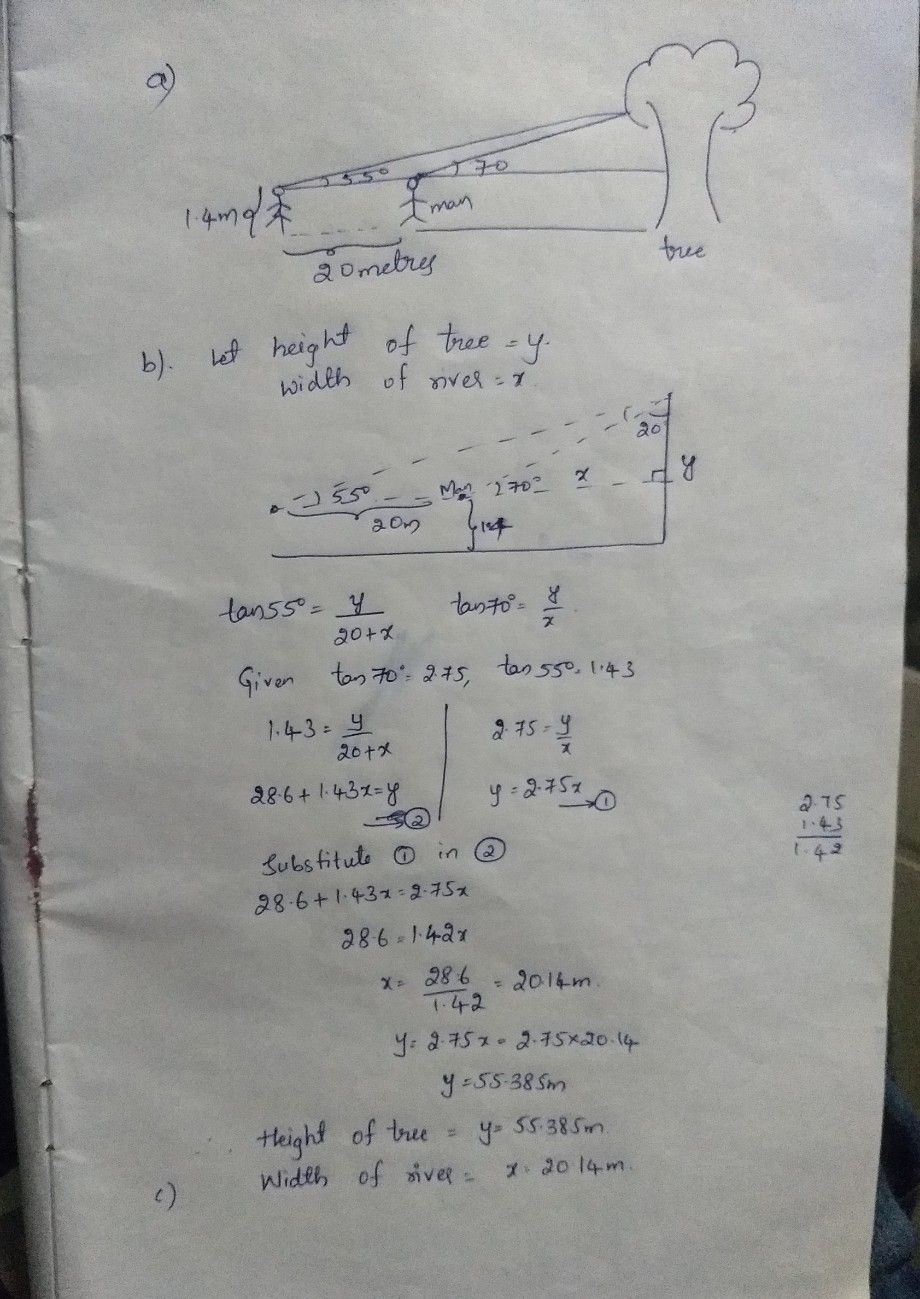Symbol
Problem$O31$ One sees the top of a tree on the bank of a river at an elevation of $70^{°}$ from the other bank. Stepping $20$ metres back, he sees the top of the tree at an elevation of $55^{°}.$ Height of the person is $1.4$ metres. $\left(a\right)$ Draw a rough figure and mark the measurements. (b) $\right)$ Find the height of the tree. $\left(c\right)$ Find the width of the river. $\left(tan70^{9}$ $=2.75:tan55^{°}$ $=1.431$ $+40$ $0$ $1$ $=$
10th-13th grade
Trigonometry
Search count: 105
SolutionQanda teacher - srikanthv